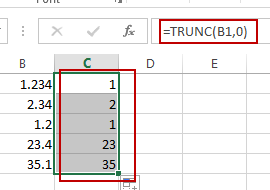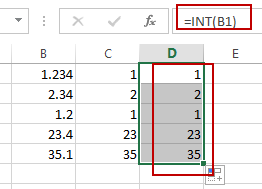# How to Delete Numbers after Decimal Point in Excel

This post will guide you how to remove decimals without rounding in Excel. How do I delete numbers after decimal point with a formula in the given cell in Excel.

## Deleting Numbers after Decimal Point

Assuming that you have a list of data in range B1:B5, in which contain decimal values. And you want to remove all decimal without rounding for a given cell in Excel. You can use the TRUNC function to achieve the result. Like this:

`=TRUNC(B1,0)`

Type this formula into a blank cell and press Enter key on your keyboard, and then drag the AutoFill Handle over to other cells to apply this formula.You can also use another formula based on the INT function to achieve the same result of deleting numbers after decimal point in Excel. Like this:

`=INT(B1)`### You might also like:

Sidebar